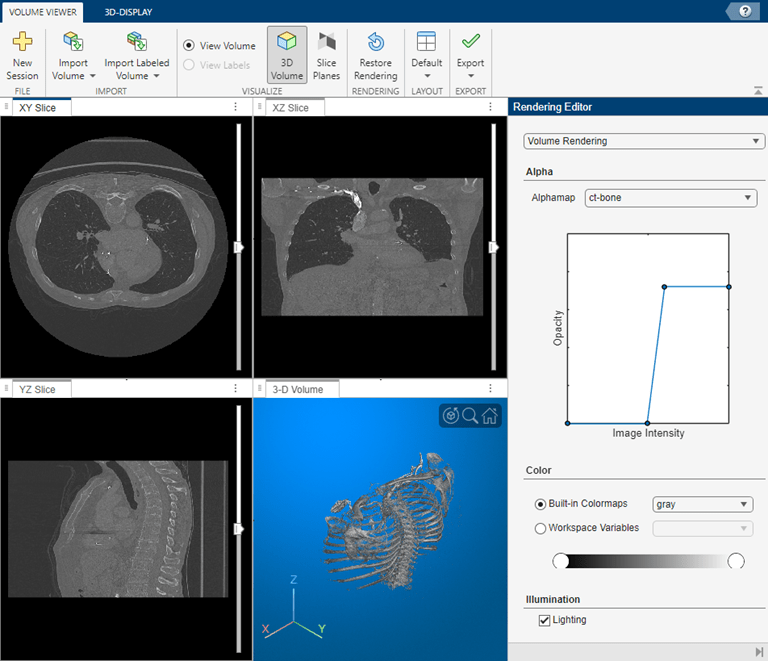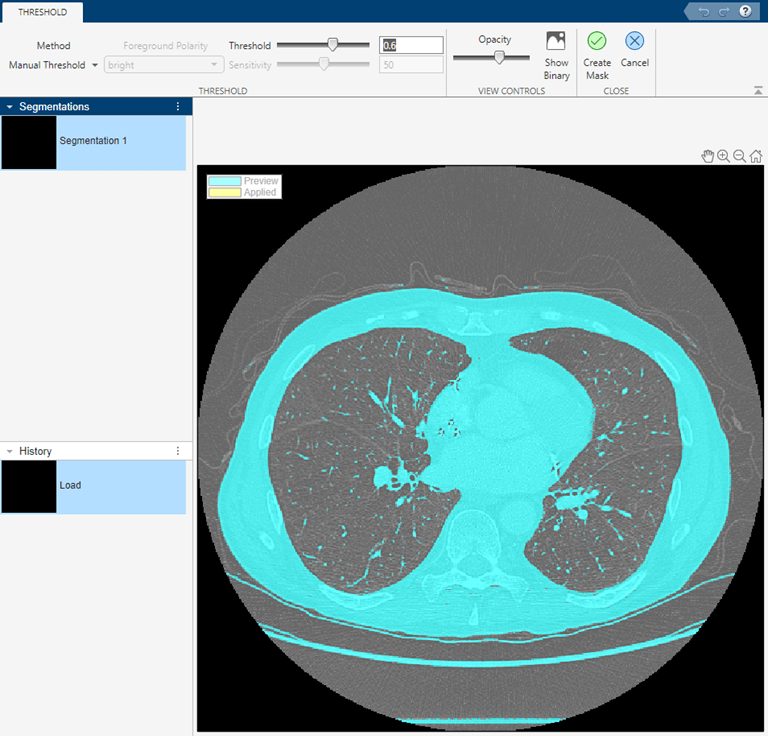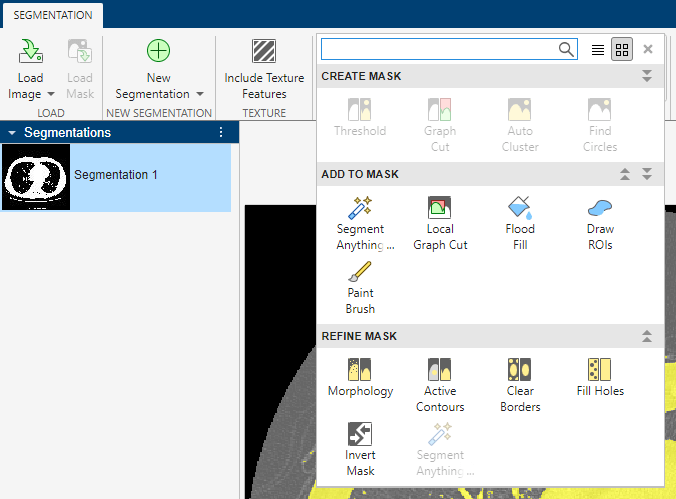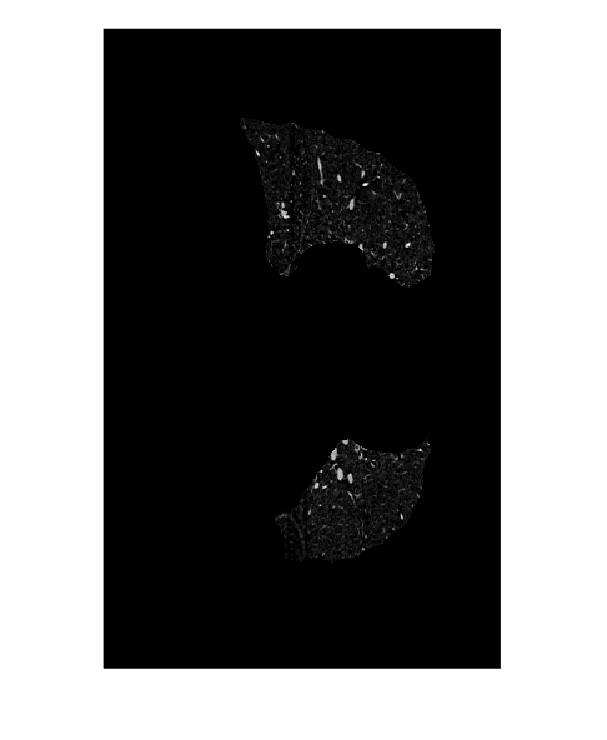Main Content

# Segment Lungs from 3-D Chest Scan

This example shows how to perform a 3-D segmentation using active contours (snakes). You can view the results using the Volume Viewer app.

### Prepare the Data

Load the human chest CT scan data into the workspace. To run this example, you must download the sample data from MathWorks™ using the Add-On Explorer. See Install Sample Data Using Add-On Explorer.

```load chestVolume whos```
``` Name Size Bytes Class Attributes V 512x512x318 166723584 int16 ```

Convert the CT scan data from `int16` to `single` to normalize the values to the range [0, 1].

`V = im2single(V);`

View the chest scans using the Volume Viewer app. Open the app from the MATLAB® Apps toolstrip. You can also open the app by using the `volumeViewer` command and specifying the volume as an argument: `volumeViewer(V)`. Volume Viewer has preset alphamaps that are intended to provide the best view of certain types of data. To get the best view of the chest scans, select the ct-bone preset.### Segment the Lungs

Segment the lungs in the CT scan data using the active contour technique. Active contours is a region growing algorithm which requires initial seed points. The example uses the Image Segmenter app to create this seed mask by segmenting two orthogonal 2-D slices, one in the XY plane and the other in the XZ plane. The example then inserts these two segmentations into a 3-D mask. The example passes this mask to the `activecontour` function to create a 3-D segmentation of the lungs in the chest cavity. (This example uses the active contour method but you could use other segmentation techniques to accomplish the same goal, such as flood-fill.)

Extract the center slice in both the XY and XZ dimensions.

```XY = V(:,:,160); XZ = squeeze(V(256,:,:));```

View the 2-D slices using the `imshow` function.

```figure imshow(XY,[],'Border','tight');````imshow(XZ,[],'Border','tight');`You can perform the segmentation in the Image Segmenter app. Open the app from the MATLAB Apps toolstrip or use the `imageSegmenter` command, specifying a 2-D slice as an argument, `imageSegmenter(XY)`.

To start the segmentation process, click Threshold to open the lung slice in the Threshold tab. On the Threshold tab, select the Manual Threshold option and move the Threshold slider to specify a threshold value that achieves a good segmentation of the lungs. Click Create Mask to accept the thresholding and return the Segmentation tab.The app executes the following code to threshold the image.

`BW = XY > 5.098000e-01;`

After this initial lung segmentation, clean up the mask using options on the Refine Mask menu.In the app, you can click each option to invert the mask image so that the lungs are in the foreground (Invert Mask), remove other segmented elements besides the lungs (Clear Borders), and fill holes inside the lung segmentation (Fill Holes). Finally, use the Morphology option to smooth the edges of the lung segmentation. On the Morphology tab, select the Erode Mask operation. After performing these steps, select Show Binary and save the mask image to the workspace.

The app executes the following code to refine the mask.

```BW = imcomplement(BW); BW = imclearborder(BW); BW = imfill(BW, 'holes'); radius = 3; decomposition = 0; se = strel('disk',radius,decomposition); BW = imerode(BW, se); maskedImageXY = XY; maskedImageXY(~BW) = 0; imshow(maskedImageXY)```Perform the same operation on the XZ slice. Using Load Image, select the `XZ` variable. Use thresholding to perform the initial segmentation of the lungs. For the XZ slice, the Global Threshold option creates an adequate segmentation (the call to `imbinarize` in the following code). As with the XY slice, use options on the Refine Mask menu to create a polished segmentation of the lungs. In the erosion operation on the Morphology tab, specify a radius of 13 to remove small extraneous objects.

To segment the XZ slice and polish the result, the app executes the following code.

```BW = imbinarize(XZ); BW = imcomplement(BW); BW = imclearborder(BW); BW = imfill(BW,'holes'); radius = 13; decomposition = 0; se = strel('disk',radius,decomposition); BW = imerode(BW, se); maskedImageXZ = XZ; maskedImageXZ(~BW) = 0; imshow(maskedImageXZ)```### Create Seed Mask and Segment Lungs Using activecontour

Create the 3-D seed mask that you can use with the `activecontour` function to segment the lungs.

Create a logical 3-D volume the same size as the input volume and insert `mask_XY` and `mask_XZ` at the appropriate spatial locations.

```mask = false(size(V)); mask(:,:,160) = maskedImageXY; mask(256,:,:) = mask(256,:,:)|reshape(maskedImageXZ,[1,512,318]);```

Using this 3-D seed mask, segment the lungs in the 3-D volume using the active contour method. This operation can take a few minutes. To get a quality segmentation, use `histeq` to spread voxel values over the available range.

```V = histeq(V); BW = activecontour(V,mask,100,'Chan-Vese'); segmentedImage = V.*single(BW);```

You can view the segmented lungs in the Volume Viewer app by running the command `volumeViewer(segmentedImage)`. By manipulating the alphamap settings in the Rendering Editor, you can get a good view of just the lungs.### Compute the Volume of the Segmented Lungs

Use the `regionprops3` function with the `'volume'` option to calculate the volume of the lungs.

`volLungsPixels = regionprops3(logical(BW),'volume');`

Specify the spacing of the voxels in the x, y, and z dimensions, which was gathered from the original file metadata. The metadata is not included with the image data that you download from the Add-On Explorer.

```spacingx = 0.76; spacingy = 0.76; spacingz = 1.26*1e-6; unitvol = spacingx*spacingy*spacingz; volLungs1 = volLungsPixels.Volume(1)*unitvol; volLungs2 = volLungsPixels.Volume(2)*unitvol; volLungsLiters = volLungs1 + volLungs2```
```volLungsLiters = 5.7726 ```

Download ebook